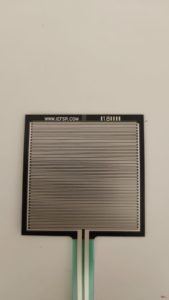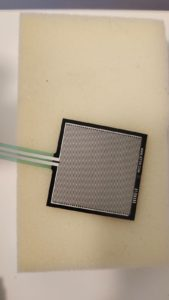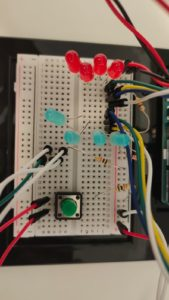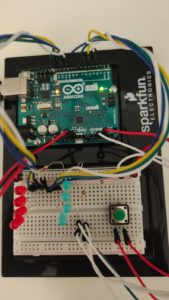# Week 9 Refill

#### Description:

I decided, after a struggle of thought, to make a simple water refill reminder. Drinking water is crucial and many people tend to try to forget. So I made this simple water that detects the weight of the bottle and knows how much water is left in the bottle/cup using a force sensor.using the force sensor on its own did not give a range of weight enough for the water weight detector. so I decided to place the force sensor on a sponge.I also used an on/off button that turns the circuit on when pressed and off when pressed once more

I also used 4 red lights that are more likely to capture someone’s attention and remind them to refill their water bottle when it’s empty.

and I used 4 blue led lights, more of the blue lights light up the more water there is in the bottle.

this is how my circuit looks:the last set of wires that are neither connected to the button or the less is connected to the force sensor.#### video:

the video shows how the circuit works:

#### Code:

```const int outs[] = {3, 5, 6, 9,
10, 11, 12, 13
};
const int ins[] = {A2, A3};
int var, var2, b, r, maxx = 0;

long timer;
bool turnon = 0, curr, prev;

void setup() {
Serial.begin(9600);
for (int i = 0; i < sizeof(outs); i++) {
pinMode(outs[i], OUTPUT);
}
Serial.begin(9600);
for (int i = 0; i < sizeof(ins); i++) {
pinMode(ins[i], INPUT);
}
}

void loop() {
if (curr != prev && curr == 1) {
turnon = !turnon;
}
timer = millis();
var = map(var, 0, 500, 0, 255);
var2 = constrain(var, 250, 255);
Serial.print(" on: ");
Serial.print(turnon);
Serial.print(" curr: ");
Serial.print(curr);
Serial.print(" prev: ");
Serial.println(prev);

if (turnon == 1) {
on();
}
else {
for (int i = 0; i < 8; i++) {
analogWrite(outs[i], 0);
}
}
//

}

void redled(int n) {
if (timer % (n * 4) < (n * 1)) {
analogWrite(outs, var2);
analogWrite(outs, 0);
analogWrite(outs, 0);
analogWrite(outs, 0);
}
else if (timer % (n * 4) < (n * 2)) {
analogWrite(outs, 0);
analogWrite(outs, var2);
analogWrite(outs, 0);
analogWrite(outs, 0);
}
else if (timer % (n * 4) < (n * 3)) {
analogWrite(outs, 0);
analogWrite(outs, 0);
analogWrite(outs, var2);
analogWrite(outs, 0);
}
else {
analogWrite(outs, 0);
analogWrite(outs, 0);
analogWrite(outs, 0);
analogWrite(outs, var2);
}
}

void on() {

if (var < 20) {
b = -1;
r = 4;
}
else if (var < 140) {
b = 0;
r = 0;
}
else if (var < 260) {
b = 1;
r = 0;
}
else if (var < 380) {
b = 2;
r = 0;
}
else {
b = 3;
r = 0;
}

// there is water
if (r == 0) {
for (int i = 0; i < b + 1; i++) {
analogWrite(outs[i], var2);
}
for (int i = 7; i > b; i--) {
analogWrite(outs[i], 0);
}
}
else {
for (int i = 0; i < 4; i++) {
analogWrite(outs[i], 0);
}
redled(1000);

}
}```